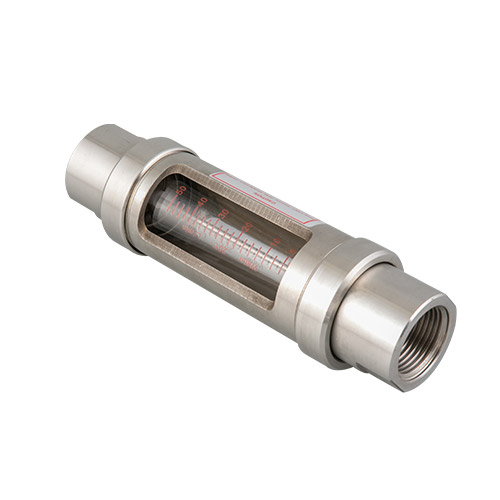###### +86-574-62503881
Inicio / News / What are the influencing factors of electromagnetic flowmeter?

# What are the influencing factors of electromagnetic flowmeter?Posted by : admin / Posted on : May 06， 2021

1. The influence of various media on measurement
⑴ The influence of flow velocity distribution is known from fluid mechanics. When the liquid flows in the pipeline, the flow velocity of each point on the cross section of the pipeline is not equal, but whether it is laminar flow or turbulent flow, after a certain distance of straight pipe, the flow velocity is divided It can become an axisymmetric distribution. The flow velocity is the largest at the center of the tube axis and zero at the tube wall. The average flow velocity is V—. As long as the flow velocity distribution is symmetrical with respect to the central axis of the measuring tube, the induced electromotive force is generated on the electrode. The size has nothing to do with the flow velocity distribution state of each point, but is only proportional to the average flow velocity of the measured liquid. Therefore, the flow velocity distribution is axisymmetric is one of the working conditions that the uniform magnetic field electromagnetic flowmeter must meet. If the flow velocity distribution is asymmetric with respect to the central axis of the tube, although the total flow is the same, the induced electromotive force near the electrode is large, so the measured signal is larger than the actual flow value. On the contrary, the induced electromotive force at a place 90° to the electrode is smaller than the actual flow value, causing measurement errors. Therefore, in order to make the flow velocity distribution axisymmetric, it is necessary to add a straight pipe section before the flowmeter.
⑵ The influence of magnetic field edge effect on measurement If it is assumed that the magnetic field is always uniform along the flow direction of the fluid, in fact, this means that the magnetic field along the tube axis is infinite, while the actual flowmeter’s magnetic field is finite. Therefore, it is necessary to consider the influence of the edge effect produced by the finite-length magnetic field on the measurement. Assuming that the tube wall is insulated, the magnetic field near the electrode is roughly uniform, and the two ends gradually weaken to form uneven edges, and finally drop to zero. In this way, the electric field E inside the liquid is not uniform, and eddy currents will be generated. The secondary magnetic flux generated by the eddy current in turn changes the working magnetic flux at the edge of the magnetic field, further destroying the uniformity of the magnetic field. At this time, the induced electromotive force measured on the electrode is different from the induced electromotive force under the infinitely long magnetic field, resulting in an error. If the tube wall is conductive, the edge effect of the magnetic field will be more obvious due to the short-circuit effect of the conductive tube wall. With the change of the tube wall conductivity and wall thickness, this effect will be more obvious, resulting in induced electromotive force on the electrode. The loss increased. For electromagnetic flowmeters, the insulation of the measuring tube wall is very necessary, so the tube wall is usually coated with an insulating layer. If the measured medium contains magnetic materials, the edge effect of the magnetic field is more complicated. Due to the presence of magnetically permeable materials, the magnetic field is severely distorted, resulting in non-linear measurement. Therefore, for liquid metal in the measured liquid, DC excitation is generally used to reduce the edge effect of the magnetic field.